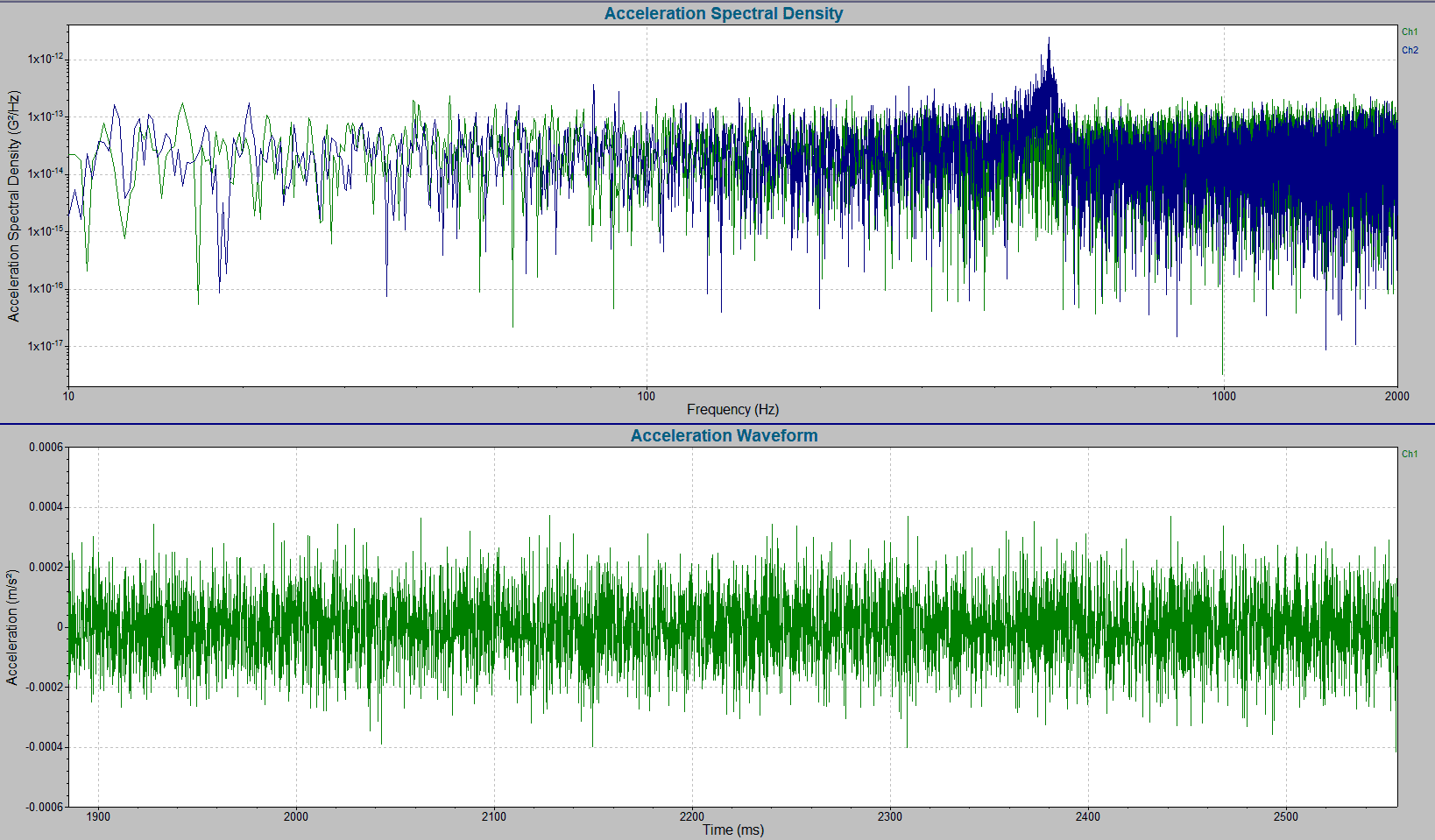# Power Spectral Density (PSD) and Random Testing

June 25, 2019

A random waveform is inherently irregular, non-deterministic, and non-repetitive. A measured point in a random time history graph cannot be used to predict any point before or after. A random waveform can be analyzed and understood from a statistical standpoint, but any specific point is unknown until it occurs.

Random waveforms are commonly used in vibration testing because they are reflective of the end-use environment. Real-world vibrational events are random and products should be tested using realistic test profiles.

### The PSD and Random Waveforms

The power spectral density (PSD) is the most common tool used to analyze random vibration. Resonances and harmonics hidden in a time-history graph are visible in a PSD graph. In practice, generating a PSD is usually the first step in examining and analyzing a random waveform.Power spectral density (above) compared to a random time-history graph (below).

Generating a useful PSD is not as simple as pushing a button. Test engineers and technicians set various parameters to tailor the PSD calculations to specific test requirements. For the parameter settings to be effective, however, the engineer must understand the PSD.

### How to Calculate the PSD

The subsequent lessons provide the steps to calculate the PSD. The lessons will communicate critical concepts without equations first and then move into a mathematically rigorous description of the PSD and related calculations.